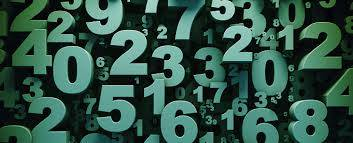what is 56/64 in simplist form?

To find out the simplest form of any fraction, you need to discover the lowest common denominator of both numbers.

This is found by dividing both numbers that make up the fraction (in this case 56 and 64) by the lowest number by which both numbers are divisible. It sounds tricky, but it's really quite easy.As both 56 and 64 are even numbers, it's obvious they can each be divided by 2:

56 divide 2 = 28

64 divide 2 = 32

So, you could say that 56/64 is the same as 28/32, and you'd be right, but the fraction can be simplified further. 28 and 32 are also divisible by 2:

28 divide 2 = 14

32 divide 2 = 16

So, we'd then have 14/16 (fourteen sixteenths). But again both numbers are divisible by 2:

14 divide 2 = 7

16 divide 2 = 8

This gives us the fraction 7/8 (seven eighths). 7 and 8 do not share 2, or any other number aside from 1, as a common denominator. Therefore, the fraction 7/8 cannot be simplified further, and the answer to your question is 56/64 written in its simplest form is 7/8.

For more Blurtit answers to questions on reducing fractions, check out What Is 2/5 In Simplest Form? and What Is 5/100 In Simplest Form?

To revise your knowledge of reducing fractions, watch this short YouTube tutorial:

thanked the writer.